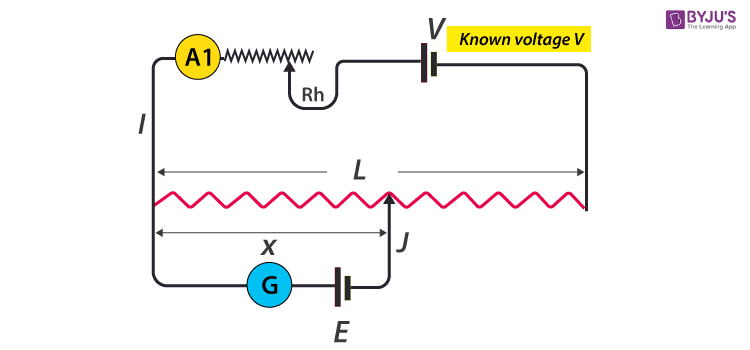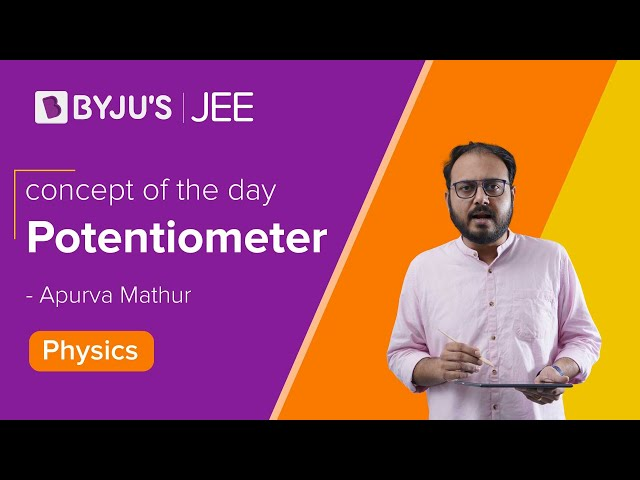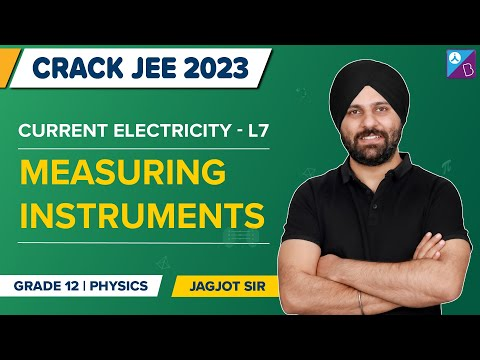Jet Set Go! All about Aeroplanes Jet Set Go! All about Aeroplanes

# Potentiometer Working

## What Is a Potentiometer?

Potentiometer working can be explained when the potentiometer is understood. It is defined as a three-terminal resistor having either sliding or rotating contact that forms an adjustable voltage divider. In order to use the potentiometer as a rheostat or variable resistor, it should have only two terminals with one end and the wiper.

Following are the terms used to describe types of potentiometers:

• Slider pot or slide pot: This can be adjusted by sliding the wiper right or left with a finger or thumb.
• Thumbwheel pot or thumb pot: This can be adjusted infrequently with the help of a small thumb wheel which is a small rotating potentiometer.
• Trimmer pot or trim pot: This can be adjusted once for fine-tuning of an electric signal.

## Potentiometer Working Principle

The potentiometer consists of L which is a long resistive wire and a battery of known EMF V, whose voltage is known as driver cell voltage. Assume a primary circuit arrangement by connecting the two ends of L to the battery terminals. One end of the primary circuit is connected to the cell whose EMF E is to be measured, and the other end is connected to galvanometer G. This circuit is assumed to be a secondary circuit.The working principle depends on the potential across any portion of the wire which is directly proportional to the length of the wire that has a uniform cross-sectional area and current flow is constant. Following is the derivation used to explain the potentiometer working principle:

$$\begin{array}{l}V=IR\end{array}$$
(Ohm’s law)

Where,

I: current

R: total resistance

V: voltage

$$\begin{array}{l}R=\frac{\rho L}{A}\end{array}$$
$$\begin{array}{l}V=I\frac{\rho L}{A}\end{array}$$

Where,

⍴: resistivity

A: cross-sectional area

With ⍴ and A constant, I is constant too for a rheostat.

$$\begin{array}{l}\frac{L\rho }{A}=K\end{array}$$
$$\begin{array}{l}V=KL\end{array}$$
$$\begin{array}{l}E=\frac{L\rho x}{A}=Kx\end{array}$$

Where,

x: length of potentiometer wire

E: cell with Lower EMF

K: constant

The galvanometer G has null detection as the potential difference is equal to zero and there is no flow of current. So, x is the length of the null point. Unknown EMF can be found by knowing x and K.

$$\begin{array}{l}E=\frac{L\rho x}{A}=Kx\end{array}$$

Since the EMF has two cells, let L1 be the null point length of the first cell with EMF E1 and L2 be the null point length of the second cell with EMF E2.

$$\begin{array}{l}\frac{E1}{E2}=\frac{L1}{L2}\end{array}$$

### Types of Potentiometer

There are basically two types of potentiometers that can be further divided depending on the movement of the wiper. Following is the table when the wiper is moving in a circular path which is known as rotary potentiometer.

 Type Description Applications Concentric pot Two potentiometers are adjusted individually with the help of concentric shafts Found in old car radios that were used to control volume and tone Single-turn pot Approximate rotation is 270 degrees in a single rotation Used where a single turn is enough to control the resolution Servo pot Used to adjust a servo motor automatically Used in remote-controls to control the volume of an audio equipment

Following is the table when the wiper is moving along a linear path which is known as linear potentiometers

 Type Description Applications Multi-turn slide A spindle is used for the construction of this kind of potentiometer Used where high precision and resolutions are required Slide pot High-quality faders are constructed using conductive plastic Used for the measurement of distance Dual-slide pot This is used to control dual slide and single slider potentiometers that are parallel Used where dual parallel channels are to be controlled

### Application of Potentiometer

• Audio control: Both linear, and rotary potentiometers, are used to control audio equipment for changing the loudness and other audio-related signals.
• Television: They are used to control the picture brightness, colour response and contrast.
• Motion control: In order to create a closed-loop control, potentiometers are used as position feedback devices known as a servomechanism.
• Transducers: As these give large output signals, they find applications in the design of displacement transducers.

Related Physics Articles:

## Videos on Potentiometer

#### Working of Potentiometer#### Measuring InstrumentsTo know more about other Physics related concepts, stay tuned with BYJU’S.

Test your Knowledge on Potentiometer Working

#### 1 Comment

1. every time i am stuck with any query byju’s always helps me thanks a lot
i hope new updates will be available for mare improvements